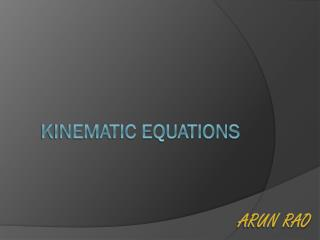# Physics Homework help - PowerPoint PPT PresentationDownload PresentationPhysics Homework help

Physics Homework helpDownload Presentation## Physics Homework help

- - - - - - - - - - - - - - - - - - - - - - - - - - - E N D - - - - - - - - - - - - - - - - - - - - - - - - - - -
##### Presentation Transcript

1. Arun Rao Kinematic equations

2. General Uses • These are used to describe the relationships between the following kinematic quantities: • Distance/displacement • Speed/velocity • Time • Acceleration • When there is an unknown, it can be solved for when the values of the other quantities are given

3. The Four Basic Kinematic Equations are: • V = V0 + a Δt • V2 = V02 + 2aΔs • S = V0Δt + 0.5 a Δt2 • S = (V0 + V)/2 × t

4. V = V0 + a Δt E.g. A car starts at rest and accelerates uniformly at 2 m/s2 for 5 seconds and stops accelerating from here on. Calculate its velocity after t = 5 seconds. Using V = V0 + a Δt, we sub in values 0 for V0, 2 for a and 5 for t. Solving for V, we get: V = 10 m/s

5. V2 = V02 + 2aΔs E.g. A train accelerates from 10 m/s to 40 m/s at an acceleration of 1m/s 2. what distance does it cover during this time. Using V2 = V02 + 2aΔs, we sub in values 40 for V, 10 for V0 and 1 for a. Re-arranging to solve for s, we get: S = 750 m

6. S = V0Δt + 0.5 a Δt2 E.g. A body starts from rest at a uniform acceleration of 3 m/s2. how long does it take to cover a distance of 100m. Using S = V0Δt + 0.5 a Δt2, we sub in values 3 for a, 0 for V0 and 100 for s. Re-arranging the equation and solving for t (using the quadratic formula), we get: t = 8.51 or -8.51 seconds. As time cannot be negative, t = 8.51 seconds.

7. S = (V0 + V)/2 × t A car decelerates from 20 m/s to 10 m/s over a period of 10 seconds. How far does it travel during this time period. Using S = (V0 + V)/2 × t, we sub in values 20 for V0, 10 for V and 10 for t. Solving for s, we get: S = 150m

8. Note: • All units must be converted such that they are uniform for different variable throughout the calculations. • Kinematic quantities that are scalar CANNOT be negative, hence any such alternate solutions obtained must be disregarded.

9. Standard units for the various quantities are as follows: • Speed – metres/second • Acceleration – metres/second squared • Distance – metres • Time - seconds

10. Thank you for listening/watching